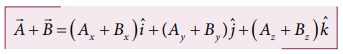Home | | Physics 11th std | Components of a Vector

# Components of a Vector

Physics : Kinematics : Components of a Vector

COMPONENTS OF A VECTOR

In the Cartesian coordinate system any vectorcan be resolved into three components along x, y and z directions. This is shown in Figure 2.20.

Consider a 3-dimensional coordinate system. With respect to this a vector can be written in component form asEXAMPLE 2.3

What are the unit vectors along the negative x–direction, negative y–direction, and negative z– direction?

Solution

The unit vectors along the negative directions can be shown as in the following figure.Then we have:

The unit vector along the negative x direction = -iˆ

The unit vector along the negative y direction = -jˆ.

The unit vector along the negative z direction = -kˆ.

In the previous section we have learnt about addition and subtraction of two vectors using geometric methods. But once we choose a coordinate system, the addition and subtraction of vectors becomes much easier to perform.

The two vectorsandin a Cartesian coordinate system can be expressed asThen the addition of two vectors is equivalent to adding their corresponding x, y and z components.Similarly the subtraction of two vectors is equivalent to subtracting the corresponding x, y and z components.The above rules form an analytical way of adding and subtracting two vectors.Tags : with Solved Example Problems , 11th Physics : UNIT 2 : Kinematics
Study Material, Lecturing Notes, Assignment, Reference, Wiki description explanation, brief detail
11th Physics : UNIT 2 : Kinematics : Components of a Vector | with Solved Example Problems